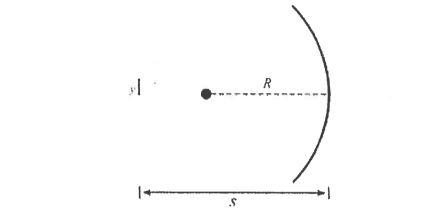# Problem: An object with height y = 2 mm is placed a distance s = 24 cm in front of the vertex of a concave spherical mirror with radius of curvature R = 16 cm, as shown in the figure below. On the figure, draw and label any two principal rays.

###### FREE Expert Solution
80% (269 ratings)
###### Problem Details

An object with height y = 2 mm is placed a distance s = 24 cm in front of the vertex of a concave spherical mirror with radius of curvature R = 16 cm, as shown in the figure below.

On the figure, draw and label any two principal rays.Frequently Asked Questions

What scientific concept do you need to know in order to solve this problem?

Our tutors have indicated that to solve this problem you will need to apply the Ray Diagrams For Mirrors concept. You can view video lessons to learn Ray Diagrams For Mirrors. Or if you need more Ray Diagrams For Mirrors practice, you can also practice Ray Diagrams For Mirrors practice problems.

How long does this problem take to solve?

Our expert Physics tutor, Juan took 1 minute and 40 seconds to solve this problem. You can follow their steps in the video explanation above.